# Parallelizing a Numpy vector Operation

• Last Updated : 18 Mar, 2022

NumPy is a library that contains multidimensional array objects as well as a collection of array processing routines. It does not operate in parallel, as you may know, however performing operations in parallel can provide us a big performance advantage. We will use numexpr library to parallelize NumPy operations.

## NumExpr

NumExpr is a fast numerical expression evaluator for NumPy. Expressions that act on the array, such as 3*a+4*b (where a and b are arrays), are accelerated and utilize less memory with it than if they were done in Python. Furthermore, its multi-threaded capabilities can utilize all of the cores, resulting in significant performance scaling as compared to NumPy.

For our needs, we’ll use the evaluate function from the numexpr package.

Syntax: numexpr.evaluate(ex, local_dict=None, global_dict=None, out=None, order=’K’, casting=’safe’, **kwargs)

Parameters:

• ex: A string forming an expression involving the operands and operators
• local_dict: A dictionary that replaces the local operands in current frame.
• global_dict: A dictionary that replaces the global operands in current frame.
• out: An existing array where the outcome is going to be stored. Care is required to ensured that the size of this array matches the resultant array size.
• order: Controls the iteration order for operands.
• casting: Controls what kind of data casting may occur when making a copy or buffering.

Returns: Resultant array

Example 1:

In this example, I have used only the ex parameter of the evaluate function to perform the addition between a and b.

## Python3

 `import` `numpy as np ` `import` `numexpr as ne ` ` `  `a ``=` `np.array([[``6``, ``6``], [``0``, ``7``]]) ` `b ``=` `np.array([[``19``, ``17``], [``13``, ``19``]]) ` ` `  `print``(ne.evaluate(``'a+b'``)) `

Output:

```[[25. 23.]
[13. 26.]]```

Example 2:

In this example, I have used the local_dict parameter, along with ex, to pass operands for c and d from the local scope.

## Python3

 `import` `numpy as np ` `import` `numexpr as ne ` ` `  `a ``=` `np.array([[``6``, ``6``], [``0``, ``7``]]) ` `b ``=` `np.array([[``19``, ``17``], [``13``, ``19``]]) ` ` `  `print``(ne.evaluate(``'c+d'``, local_dict``=``{``'c'``: a, ``'d'``: b})) `

Output:

```[[25. 23.]
[13. 26.]]```

Example 3:

In this example, I have used global_dict parameter to pass operand for d whereas the local_dict parameter to pass operand for c in the ex parameter.

## Python3

 `import` `numpy as np ` `import` `numexpr as ne ` ` `  `a ``=` `np.array([[``6``, ``6``], [``0``, ``7``]]) ` ` `  `def` `calc(): ` `    ``b ``=` `np.array([[``19``, ``17``], [``13``, ``19``]]) ` `    ``out ``=` `np.zeros((``2``, ``2``)) ` `    ``ne.evaluate(``'c+d'``, local_dict``=``{``'c'``: b}, ` `                ``global_dict``=``{``'d'``: a}, out``=``out) ` `    ``print``(out) ` ` `  `calc()`

Output:

```[[25. 23.]
[13. 26.]]```

## Comparing Performance

Now, let us put our learning to the test now. In the following examples, we will perform the same operations using both NumPy and NumExpr and timed them using timeit.

Example 1:

We will perform a simple algebraic expression involving addition and multiplication on two-row vectors, each of size 1000000. For the sake of doing that, we have used add and multiply functions from the NumPy package and evaluate from NumExpr to mimic it.

## Python3

 `import` `numpy as np ` `import` `numexpr as ne ` ` `  `a ``=` `np.arange(``1000000``) ` `b ``=` `np.arange(``1000000``) ` ` `  `timeit np.add(np.multiply(``2``, a), np.multiply(``4``, b)) ` `timeit ne.evaluate(``'2*a+4*b'``) `

Output: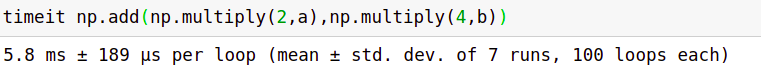Output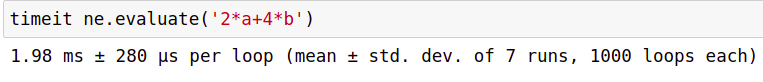Output

Example 2:

In this example, we will use the sin function from the NumPy library and its counterpart using NumExpr’s evaluate a function on a 1000000-sized row vector.

## Python3

 `import` `numpy as np ` `import` `numexpr as ne ` ` `  `a ``=` `np.arange(``1000000``) ` ` `  `timeit np.sin(a) ` `timeit ne.evaluate(``'sin(a)'``) `

Output: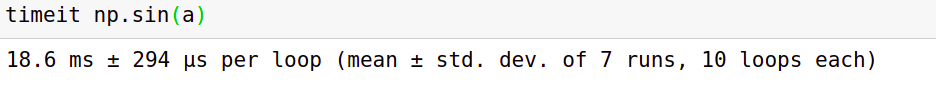Output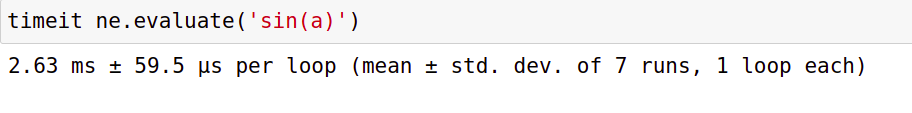Output

Example 3:

In this example, we will use the cos function from the NumPy library and its counterpart using NumExpr’s evaluate function on a (10000, 10)-sized matrix.

## Python3

 `import` `numpy as np ` `import` `numexpr as ne ` ` `  `a ``=` `np.random.randint(``0``, ``1000``, size``=``(``10000``, ``10``)) ` ` `  `timeit np.cos(a) ` `timeit ne.evaluate(``'cos(a)'``) `

Output: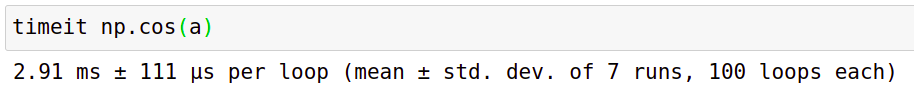Output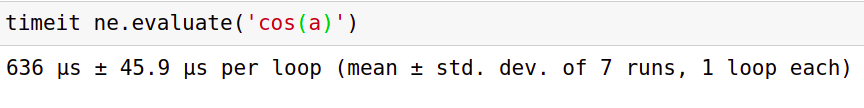Output

My Personal Notes arrow_drop_up
Recommended Articles
Page :